# Parallelogram

In the parallelogram we know one internal angle 67°33`. Calculate the other internal angles.

β =  112.45 °
γ =  67.55 °
δ =  112.45 °

### Step-by-step explanation:

$\beta =180-\left(67+33\mathrm{/}60\right)=112.45\text{°}=112\mathrm{°}2{7}^{\mathrm{\prime }}$
$\gamma =67+33\mathrm{/}60=67.55\text{°}=67\mathrm{°}3{3}^{\mathrm{\prime }}$
$\delta =180-\left(67+33\mathrm{/}60\right)=112.45\text{°}=112\mathrm{°}2{7}^{\mathrm{\prime }}$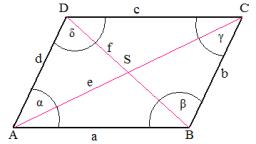Did you find an error or inaccuracy? Feel free to write us. Thank you!#### You need to know the following knowledge to solve this word math problem:

We encourage you to watch this tutorial video on this math problem:

## Related math problems and questions:

• TrianglesFind out whether given sizes of the angles can be interior angles of a triangle: a) 23°10',84°30',72°20' b) 90°,41°33',48°37' c) 14°51',90°,75°49' d) 58°58',59°59',60°3'
• AnglesThe triangle is one outer angle 158°54' and one internal angle 148°. Calculate the other internal angles of a triangle.
• 3-bracket 3Two angles in a triangle are 90° and 60°. Has triangle at least two equal sides?
• Find theFind the third interior angle of the triangle ABC where: α = 48°, γ = 65°.
• Two anglesThe triangles ABC and A'B'C 'are similar. In the ABC triangle, the two angles are 25° and 65°. Explain why in the triangle A'B'C 'is the sum of two angles of 90 degrees.
• Rhombus anglesIf one angle in the rhombus is 159°, what is its neighboring angle in rhombus?
• Complementary angles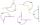Two angles are complementary. If one angle measures 10°, what is the measure of the second angle?
• SimilarityAre two right triangles similar to each other if the first one has an acute angle 70°, and the second one has an acute angle 20°?
• Angles in triangle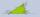Calculate the alpha angle in the triangle if beta is 61 degrees and 98 gamma degrees.
• Clocks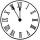What distance will describe the tip of minute hand 6 cm long for 20 minutes when we know the starting position with finally enclose hands each other 120°?
• The two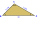The two angles of a triangle are 78° and 82°. So what is the measure of the remaining third angle?
• RWYCalculate the opposite direction of the runway 13. Runways are named by a number between 01 and 36, which is generally one tenth of the azimuth of the runway's heading in degrees: a runway numbered 09 points east (90°), runway 18 is south (180°), runway 2
• Gamma angleFind the magnitude of the gamma angle in triangle ABC if: α = 38° 56 ’and β = 47° 54’.
• Supplementary angles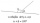One of the supplementary angles is larger by 33° than the second one. Calculate the angles size.
• Diamond and anglesFind the area of a diamond with a side of 5 cm if you know that the internal angles in the diamond are 60° and 120°.
• MO Z7–I–6 2021In the triangle ABC, point D lies on the AC side and point E on the BC side. The sizes of the angles ABD, BAE, CAE and CBD are 30°, 60°, 20° and 30°, respectively. Find the size of the AED angle.
• Internal anglesOne internal angle of the triangle JAR is 25 degrees. The difference is the size of the two others is 15°. Identify the size of these angles.